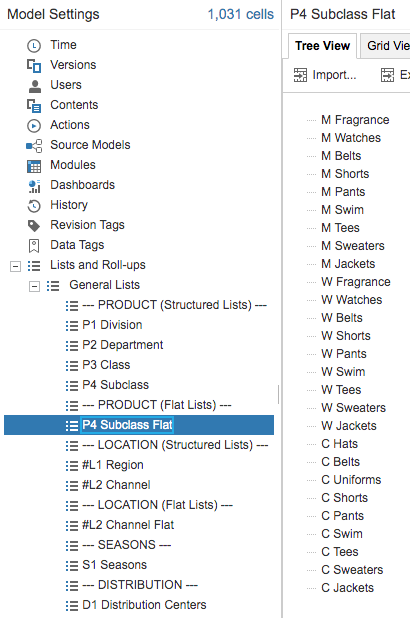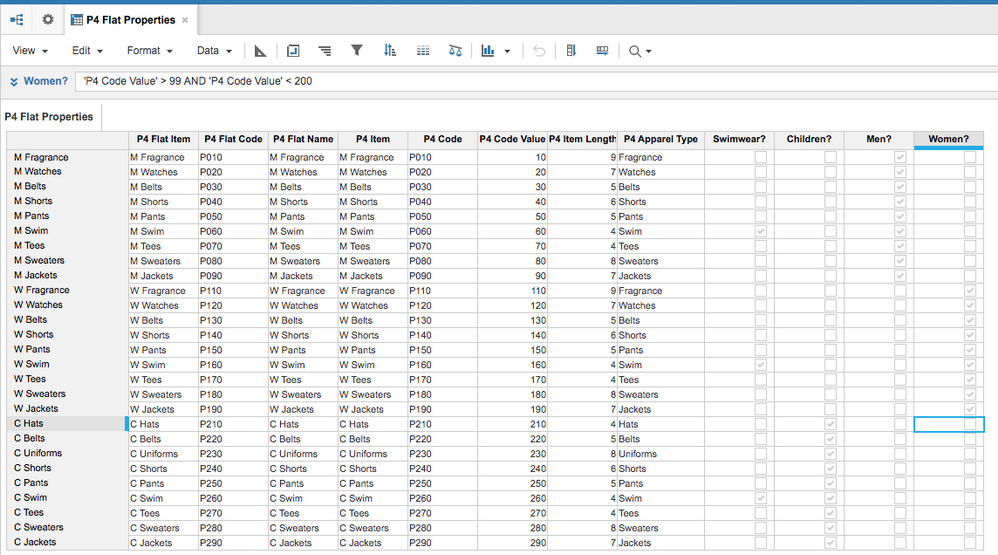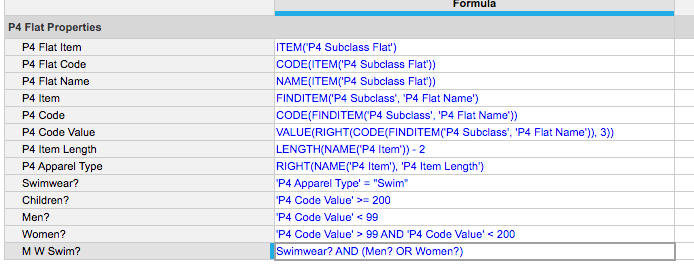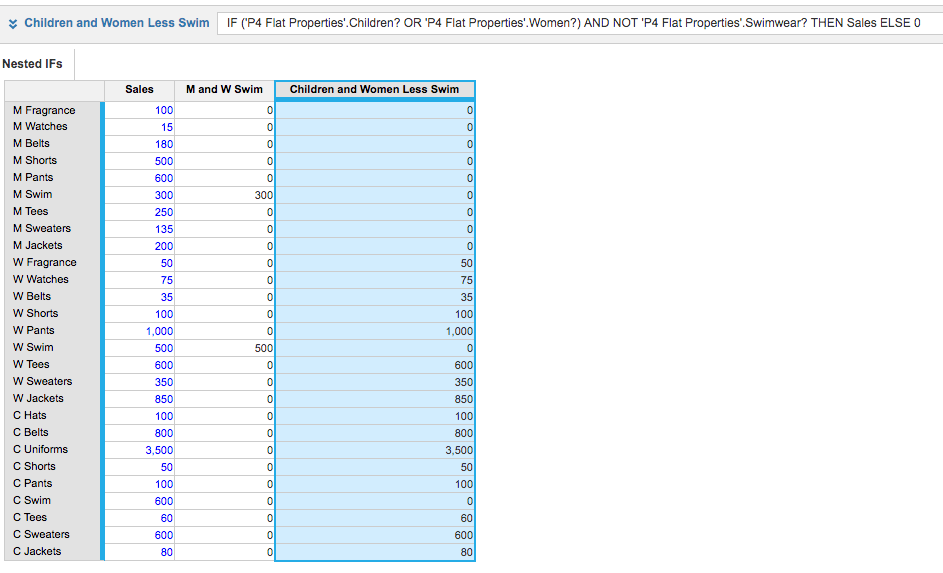# Formula AssistanceI am trying to build a formula to pull in fringe benefit rates based on the department/unit and employee works for. I attempted to build an IF Then formula but Anaplan keeps telling me it is invalid. See my formula below.

IF Dept Code >= 0000 and Dept Code <= 5999 OR Dept Code = 6500 OR >= 7000 and Dept Code <=7199 THEN UPG Admin FB Rates.Fringe Rate[LOOKUP: Staff Types (UPG)] OR IF Dept Code >= 6000 and Dept Code <= 6999 THEN UPG CPG Fringe Rates.Fringe Rates[LOOKUP: Staff Types (UPG)] OR IF Dept Code >= 8700 and Dept Code <= 8799 THEN UPG RPCN Fringe Rates.Fringe Rate[LOOKUP: Staff Types (UPG)] OR IF Dept Code >= 8000 and Dept Code <= 8600 THEN UPG RPCS Fringe Rates.Fringe Rate[LOOKUP: Staff Types(UPG)] OR ELSE 0

Any assistance is appreciated.

Thanks,

Davrae

Tagged:

•I would strongly suggest you build a system module for departments and use booleans in your calculation. Check out the DISCO methodology.

This will make your if statement easier to read and be more efficient.

For example, you would create a system module for your department list. Only departments would be in this list (so make it flat - no parents or descendants). Then add line items that help you filter the departments. For instance, if the department code is less than 5999 then "TRUE".

Lastly, it is recommended that if you have a lot of IF, Then, Else, nested together you break them up.

Let me know if this is enough detail. I can create an example for you.

•Here's an example which also demonstrates the PLAN methodology and DISCO.

First we create a flat list from our structured list that only contains that hierarchy level. In this case we'll focus on the the subclass, our lowest level (this could be your department in your example)Following DISCO, or the "S" for System, we create a system module using only the flat list we just created.

This will contain all our booleans for filtering and for nested IF statements.

In the PLAN methodology, this would be the "P" for performance and "A" for auditing.

Notice we create all our booleans here, even the complicated ones. "Calculate Once, Reuse Often"Here are the formulas for our system module. Very simple, concise, and easy to understand what they do.Lastly, we create an Output module, or "O" in DISCO.

Several things to note here:

- The nested if statement is very understandable "A", and uses the booleans from the system module. Very fast ("P")!

- Always set up your IF statements to calculate the most likely scenario first. For example, most of the subclasses for our sales for the C and W less Swim sales (below) will meet this condition, so we test for that first.Hope this helps!

•Hello Jared,

I am going to have to study this some as I am not following the example. I am not comfortable making any changes or adding to the hierarchy, as our implementation consultants managed the hierarchy and we were not trained on the process. I  will try to study this some more and see what I can come up with.

•Hi Davrae,
It looks like the logic may need adjustment. Try this.
IF Dept Code >= 0000
AND Dept Code <= 5999
OR Dept Code = 6500
OR >= 7000
AND Dept Code <=7199
THEN UPG Admin FB Rates.Fringe Rate[LOOKUP: Staff Types (UPG)]
ELSE IF Dept Code >= 6000
AND Dept Code <= 6999
THEN UPG CPG Fringe Rates.Fringe Rates[LOOKUP: Staff Types (UPG)]
ELSE IF Dept Code >= 8700
AND Dept Code <= 8799
THEN UPG RPCN Fringe Rates.Fringe Rate[LOOKUP: Staff Types (UPG)]
ELSE IF Dept Code >= 8000
AND Dept Code <= 8600
THEN UPG RPCS Fringe Rates.Fringe Rate[LOOKUP: Staff Types(UPG)]
ELSE 0
•Hi,

I second Jared here. You don't need to make any change really.

Just create a module with department as its only dimension and set a fringe rate for every department

Module Name.Fringe Rate[LOOKUP:my department]

where my department is a department list formated line item (that you will probbly obtain with FINDITEM)

•HI @davraes,

I was going thru your formula and found some issues. Please find the corrected formula below. Let me know if that works.

IF (Dept Code >= 0000 and Dept Code <= 5999) OR (Dept Code = 6500) OR (Dept Code>= 7000 and Dept Code <=7199) THEN UPG Admin FB Rates.Fringe Rate[LOOKUP: Staff Types (UPG)] Else IF (Dept Code >= 6000 and Dept Code <= 6999) THEN UPG CPG Fringe Rates.Fringe Rates[LOOKUP: Staff Types (UPG)] Else IF (Dept Code >= 8700 and Dept Code <= 8799) THEN UPG RPCN Fringe Rates.Fringe Rate[LOOKUP: Staff Types (UPG)] Else IF (Dept Code >= 8000 and Dept Code <= 8600) THEN UPG RPCS Fringe Rates.Fringe Rate[LOOKUP: Staff Types(UPG)] ELSE 0

Regards,

Kavin.

•First its invalid because there is no logic in the formula and you need to add few more paranthesis here and there as @kavinkumar suggested.

However @JaredDolich has already given you much more simpler, faster and effective approach of doing it.

Happy Planning!

Misbah

•First impression here is that you formula is far to long. Break out your formula across more line items.

Secondly, you are using hard coded numbers which really should be coming from an input module or a list. From the nature of what you appear to be trying to do the Dept Code should be a list. This should either be a property of the employee list or a list formatted line item in a system module for employee details. Create an input module containing the department code list as a dimension and benefit rate as a line item. In your target module which should be dimensioned by the employee list use LOOKUP to pull the rate through per employee dependent on the department. The LOOKUP should reference the department property of the employee list or list formatted line item of the employee details module.

There are a few steps required but in summary;

1 Create a new list of department codes.
2 Create a property of the employee list or list formatted line item in an employees details system module.
3 Create a new module containing the departments list containing one line item contain the fringe benefit rate
4 Use LOOKUP in your target module to take the rate relevant to the employees department querying the employee list property or list formnatted line item

Where you are trying to build a formula with more than two queries this should raise alarm bells and prompt you to seek out a simpler solution.

Hope this helps.

Chris
•Hello Jared,

I read over your response over the weekend and it made more sense to me. My concern is the flat list. Our implementation team built a flat list in the Hub and I am not sure how to move that list from the Hub to Development. Maybe I need to created a flat list in DEV.   Your thoughts?

Thank you,

Davrae

•Hello AMW ,

Thank you for your assistance. Anaplan did not like this formula.